# Quantum and Survey Reporter t-Test of a Proportion

Where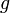$g$ is the observed proportion and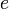$e$ is the expected proportion and$n$ is the effective sample size, the test statistic is: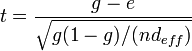$t = \frac{g - e}{\sqrt{g(1-g)/(nd_{eff})}}$
where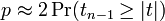$p \approx 2\Pr(t_{n-1} \ge |t|)$ if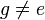$g \ne e$ and NaN otherwise, and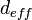$d_{eff}$ is Extra deff.